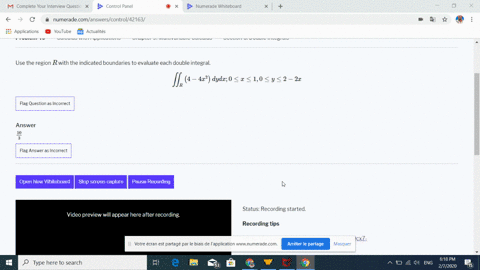Enroll in one of our FREE online STEM bootcamps. Join today and start acing your classes!View Bootcamps### Use the region $R$ with the indicated boundaries …

11:39Georgia Southern University
Problem 48

# Use the region $R$ with the indicated boundaries to evaluate each double integral.$$\iint_{R}(2 x+6 y) d y d x ; \quad 2 \leq x \leq 4,2 \leq y \leq 3 x$$

## Discussion

You must be signed in to discuss.

## Video Transcript

Okay, so we have this double Integral. Two, two, four, and then two to three effects. James. Trade three eggs, actually. Okay. And then to x six y deride X start with an anti derivative with respect. Why? So it's going to give us Teo X Y plus three. I squared. Okay. And we need to evaluate this. Who from, like ALS to try elsewhere? No. Michael's two to three eggs, brother. Okay, so two to four. This is gonna be to X times. Okay, three X minus two, and then plus three times nine x squared. Minus for Hey, so let's go ahead and distribute all this out. This is six X squared, minus four acts. What is THISS twenty seven X, uh, squared. My twelve. Okay. So anti derivative of this will be X go so divided by T to execute minus two x squared. And then that'LL be plus nine. Execute minors. Talk Max. Evaluated from two to four. This will be to times for cubed minus two cubes of sixty four minus eight. Okay, that is two times sixteen minus full. Kirk plus nine times sixty form and say minus twelve times. Uh, four minus two Okay, Two times. Fifty six. That's one twelve Minus two times twelve. Twenty four, nine times fifty six. Okay, ten times fifty six is five. Sixty and announce. Attract one fifty six. So that'LL be what? Five. Oh, for and then minus another twenty four. Okay, so one twelve and five o four. That's six sixteen. And then minus forty eight. Yeah, that should be five sixty eight.